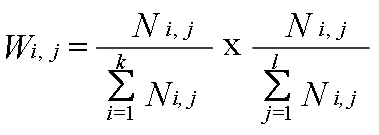Tumor Associated Gene

 Establishment of weight matrix for TAG domain     The two dimensional term frequency (2DTF) formula is adopted from the tfidf weight (term frequencyinverse document frequency) which is a weight often used in information retrieval and text mining. The tfidf weight is a statistical measure used to evaluate how important a word is to a document. The importance increases proportionally to the number of times a word appears in the document (i.e., tf) but is offset by how common the word is in all of the documents in the collection (i.e., df). In our case, we dont take df offset from the tf to remove the commoness. Alternatively, the tf is modified by the relative importance of the given term in multiple document in the collection. That is, we multiply the term frequencies from two dimentions to calculate a final weight score for the relative importance of a given term in a document.     The term frequency in the given document gives a measure of the importance of the term ti within the particular document.In our case, ni being the number of occurrences of a given protein domain, and the denominator is the number of occurrences of all protein domain in the oncogene or TSG groups.     Then the two dimensional Term Frequency (2DTF) formula isWhere N i,j being the number of occurrences of a given protein domain, and the denominator is the number of occurrences of all protein domain in the oncogene or TSG groups.     A high weight in 2DTF is reached by a high term frequency in the given category and a low term frequency across mutiple categories; the weights hence tends to enhance the relative importance of terms in one category verses the other. A weight matrix table was generated based on calculated score for each protein domain and the oncogenic potenial of any protein is the score sum from all domains present in the given protein.

 TAG web site designed and supported by Dillon Chan.